# Math in Focus Grade 2 Chapter 3 Practice 9 Answer Key Subtraction Across Zeros

Go through the Math in Focus Grade 2 Workbook Answer Key Chapter 3 Practice 9 Subtraction Across Zeros to finish your assignments.

## Math in Focus Grade 2 Chapter 3 Practice 9 Answer Key Subtraction Across Zeros

Regroup.
Then subtract.

Question 1.
200 – 45 = ?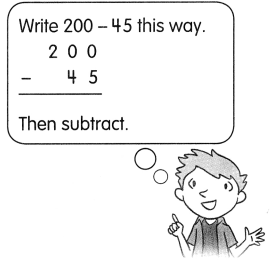200 = 2 hundreds
= 1 hundred _________ tens
= 1 hundred ________ tens 10 ones
200 – 45= _______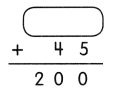200-45 = 155.
155+45 = 200.

Explanation:
Here, 200-45 is 155. As
200 = 2 hundreds
= 1 hundred 10 tens
= 1 hundred 9 tens 10 ones.
So 200-45 = 155.
155+45 = 200.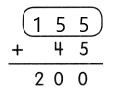Question 2.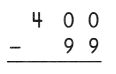400-99 = 301,
301+99 = 400.

Explanation:
The subtraction of 400-99 is 301. So to check the answer we will perform an addition which is 301+99 = 400.

Question 3.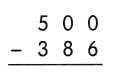500-386 = 114,
114+386 = 500.

Explanation:
The subtraction of 500-386 is 114. So to check the answer we will perform an addition which is 114+386 = 500.

Question 4.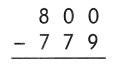800-779 = 21,
779+21 = 800.

Explanation:
The subtraction of 800-779 is 21. So to check the answer we will perform an addition which is 779+21 = 800.

Question 5.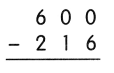600-216 = 384,
384+216 = 600.

Explanation:
The subtraction of 600-216 is 384. So to check the answer we will perform an addition which is 384+216 = 600.

Question 7.
900 – 789 = ___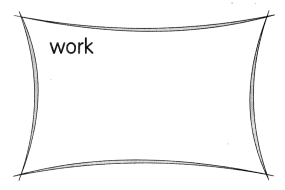900-789 = 111,
111+789 = 900.

Explanation:
The subtraction of 900-789 is 111. So to check the answer we will perform an addition which is 111+789 = 900.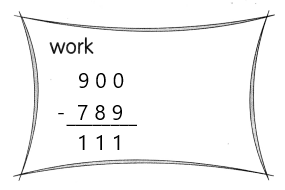Solve.

Question 8.
700 children enter an art contest. 98 of them win a prize. How many children do not win a prize?
____ children do not win a prize.
602 children do not win a prize.

Explanation:
Given that 700 children enter an art contest and 98 of them win a prize. So the number of children who do not win a prize is 700-98 which is 602 children.

Question 9.
The library needs to order 600 books. It has ordered 263 books. How many more books does the library still need to order?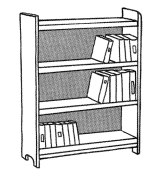The library still needs to order ___ more books.